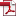• KSII Transactions on Internet and Information Systems
Monthly Online Journal (eISSN: 1976-7277)

# Optimal Base Station Clustering for a Mobile Communication Network Design

Vol. 5, No. 5, May 30, 201110.3837/tiis.2011.05.011, Download Paper (Free):#### Abstract

This paper considers an optimal base station clustering problem for designing a mobile (wireless) communication network. For a given network with a set of nodes (base stations), the problem is to optimally partition the set of nodes into subsets (each called a cluster) such that the associated inter-cluster traffic is minimized under certain topological constraints and cluster capacity constraints. In the problem analysis, the problem is formulated as an integer programming problem. The integer programming problem is then transformed into a binary integer programming problem, for which the associated linear programming relaxation is solved in a column generation approach assisted by a branch-and-bound procedure. For the column generation, both a heuristic algorithm and a valid inequality approach are exploited. Various numerical examples are solved to evaluate the effectiveness of the LP (Linear Programming) based branch-and-bound algorithm.